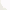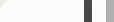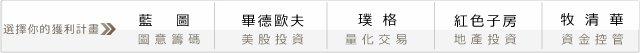## 坊間大絕之技術分析－KD指標KD的計算方式如下：

RSV ＝（第n天收盤價-最近n天內最低價）/（最近n天內最高價-最近n天內最低價）×100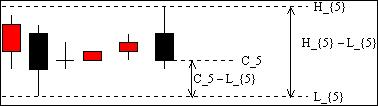#### 新手教室

n是我們可以去改變的數值，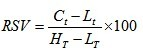RSV=((close-lowest(low,Length))
/(highest(high,Length)-lowest(low,Length)))*100;

KD值的公式如下：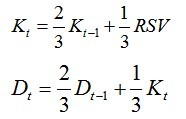Input:Length(9),Up(70),Dn(30);
Var:RSV(0),K_V(50),D_V(50),x(0);

if CurrentBar=Length then begin
K_V=50;
D_V=50;
end;

If CurrentBar >Length AND Length > 0 Then Begin
if highest(high,Length)<>lowest(low,Length) then begin
RSV=((close-lowest(low,Length))/(highest(high,Length)-lowest(low,Length)))*100;
K_V=RSV/3+(2*K_V)/3;
D_V=K_V/3+(2*D_V)/3;
end;
End;

Plot1(K_V, "%K",red);
Plot2(D_V, "%D",green);
plot3(Up, "up",blue);
plot4(Dn, "dn",blue);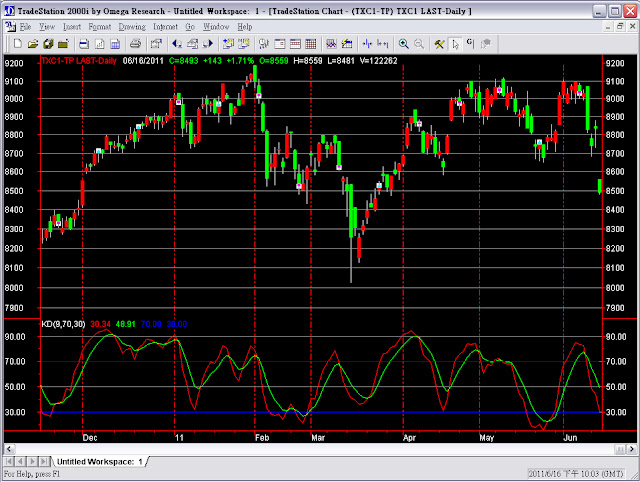#### 1 意見: